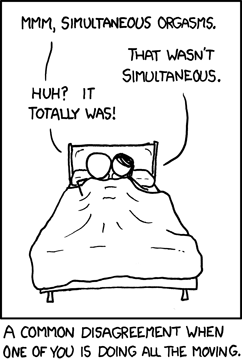# Simultaneity Bias: Simple Definition

Regression Analysis > Simultaneity Bias

## What is Simultaneity?

Simultaneity is where the explanatory variable is jointly determined with the dependent variable. In other words, X causes Y but Y also causes X. It is one cause of endogeneity (the other two are omitted variables and measurement error).

A similar (and often confused) bias is reverse causation, where Y causes X (but X does not cause Y).

## A Non-Technical Definition

A simple (non-technical) example can be found in the social sciences. Merton(1968) first wrote about simultaneity and social status: high-status intellectuals get more credit for comparable achievements than lower-status colleagues — resulting in more than their fair share of the rewards. This collection of rewards and accolades means that their statuses are amplified even further, leading to even larger advantages. This feedback loop is sometimes called the “Matthew Effect” or “cumulative advantage.”

An even more basic example from XKCD :## What Causes It?

Simultaneity happens when two variables on either side of a model equation influence each other at the same time. In other words, the flow of causality isn’t a hundred percent from a right hand side variable (i.e. a response variable) to a left hand side variable (i.e. an explanatory variable). It could be that:

• Changes in a right hand side variable are causing changes in a left hand side variable.
• Variables on the left hand side and right hand side are jointly determined.

Models with simultaneity are called simultaneous equations models or structural models. SEM theory is specifically set up to deal with the potential for endogeneity in a regression model.

## What is Simultaneity Bias?

Simultaneity bias is a term for the unexpected results that happen when the explanatory variable is correlated with the regression error term, ε (sometimes called the residual disturbance term), because of simultaneity. It’s so similar to omitted variables bias that the distinction between the two is often very unclear and in fact, both types of bias can be present in the same equation.

The standard way to deal with this type of bias is with instrumental variables regression (e.g. two stage least squares).

References:
Merton, R. K. 1968. The Matthew Effect in science. Science 159(3810) 56–63.
Wooldridge, Jeffrey M. (2013). Introductory Econometrics: A Modern Approach (Fifth international ed.). Australia: South-Western. pp. 82–83.

CITE THIS AS:
Stephanie Glen. "Simultaneity Bias: Simple Definition" From StatisticsHowTo.com: Elementary Statistics for the rest of us! https://www.statisticshowto.com/simultaneity-bias/